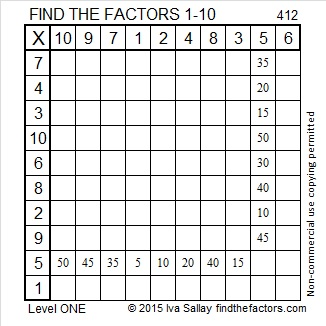# 412 and Level 1

Because 12 is divisible by 4, we know that 412 is also divisible by 4.

The number 12 has six factors. Does 412 have more than that? Find out after the puzzle!Print the puzzles or type the factors on this excel file: 10 Factors 2015-03-02

• 412 is a composite number.
• Prime factorization: 412 = 2 x 2 x 103, which can be written 412 = (2^2) x 103
• The exponents in the prime factorization are 2 and 1. Adding one to each and multiplying we get (2 + 1)(1 + 1) = 3 x 2  = 6. Therefore 412 has exactly 6 factors.
• Factors of 412: 1, 2, 4, 103, 206, 412
• Factor pairs: 412 = 1 x 412, 2 x 206, or 4 x 103
• Taking the factor pair with the largest square number factor, we get √412 = (√4)(√103) = 2√103 ≈ 20.2978

Surprised?This site uses Akismet to reduce spam. Learn how your comment data is processed.